# Examples On Evaluating Limits Set-1

Go back to  'LCD'

## Examples On Evaluating Limits

Example - 1

Evaluate the following limits

\begin{align}&{\rm\bf{(a)}}\quad \mathop {\lim }\limits_{x \to 2} \left( {\frac{2}{{x(x - 2)}} - \frac{1}{{{x^2} - 3x + 2}}} \right) \qquad \qquad {\rm\bf{(b)}}\quad \mathop {\lim }\limits_{n \to \infty } \left[ {(1 + x)(1 + {x^2})(1 + {x^4})....(1 + {x^{{2^n}}})} \right]\,\,\,|x|\, < 1\\ &{\rm\bf{(c)}} \quad \mathop {\lim }\limits_{x \to 3} \,\,\,\frac{{{x^3} - 7{x^2} + 15x - 9}}{{{x^4} - 5{x^2} + 27x - 27}}\end{align}

Solution: (a) This limit is of the indeterminate form $$\infty - \infty$$ . Combining the two fractions in this limit should lead to a cancellation of the factor giving rise to this indeterminacy, i.e. (x – 2)

$\mathop {\lim }\limits_{x \to 2} \,\left( {\frac{2}{{x(x - 2)}} - \frac{1}{{{x^2} - 3x + 2}}} \right)\,\,$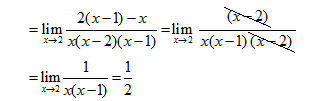(b) Before trying to solve this, try to feel that this expression will have a finite limit even though the number of factors being multiplied tends to infinity. This is because the successive factors become closer and closer to 1 and their ‘contribution’ to the final product becomes smaller and smaller Now, to simplify this product, we multiply it by \begin{align}\frac{{1 - x}}{{1 - x}}.\end{align} This is what happens: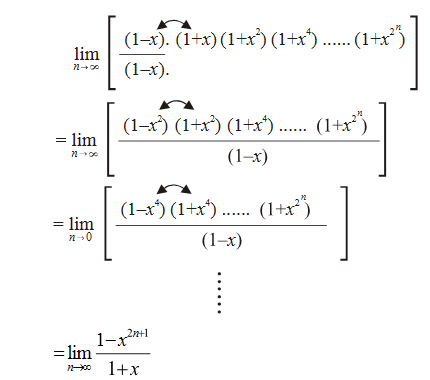Since $$|x|\,\, < 1,\;\mathop {\lim }\limits_{n \to \infty } {x^{{2^{n + 1}}}} = 0$$

Hence, the value of the limit is $$\frac{1}{{1 - x}}$$

(c) The numerator and denominator both tend to 0 as $$x \to 3$$ because of the common factor (x – 3).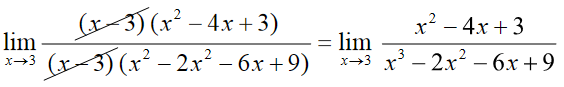There is still another common (x – 3) left in both the numerator and the denominator.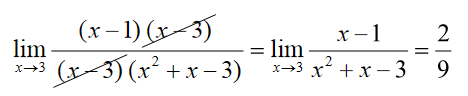Example - 2

Evaluate the following limits:

 $\;(a)\quad \mathop {\lim }\limits_{x \to 4} \frac{{\sqrt {1 + 2x} - 3}}{{\sqrt x - 2}}$ $\;(b)\quad \mathop {\lim }\limits_{x \to 1} {\mkern 1mu} {\mkern 1mu} \frac{{{x^2} - \sqrt x }}{{\sqrt x - 1}}$ $\;(c)\quad {\rm{}}\mathop {\lim }\limits_{x \to \infty } {\mkern 1mu} {\mkern 1mu} \frac{{\sqrt {{x^2} + 1} - \sqrt{{{x^2} + 1}}}}{{\sqrt{{{x^4} + 1}} - \sqrt{{{x^4} + 1}}}}$ $\;(d)\quad \mathop {\lim }\limits_{x \to 1} {\mkern 1mu} {\mkern 1mu} \frac{{(x + {x^2} + ...... + {x^n}) - n}}{{x - 1}}$

Solution: (a) This limit can evidently be solved by rationalising both the numerator and the denominator.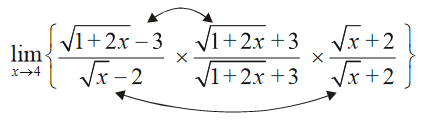\begin{align} &= \mathop {\lim }\limits_{x \to 4} \left\{ {\frac{{2x - 8}}{{x - 4}}\,\,\,\, \times \,\,\,\,\frac{{\sqrt x + 2}}{{\sqrt {1 + 2x} + 3}}} \right\} \\ &= 2\mathop {\lim }\limits_{x \to 4} \frac{{\sqrt x + 2}}{{\sqrt {1 + 2x} + 3}} \\ &= \frac{4}{3} \\ \end{align}

(b) This can be solved by rationalisation again.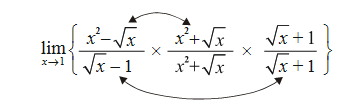\begin{align} &= \mathop {\lim }\limits_{x \to 1} \left\{ {\frac{{{x^4} - x}}{{x - 1}}\,\,\,\, \times \,\,\,\,\,\frac{{\sqrt x + 1}}{{{x^2} + \sqrt x }}} \right\} \\& = \mathop {\lim }\limits_{x \to 1} \left\{ {x\left( {{x^2} + x + 1} \right)\,\,\,\, \times \,\,\,\,\frac{{\sqrt x + 1}}{{{x^2} + \sqrt x }}} \right\} \\ &= 3\\ \end{align}

(c) This limit is of the indeterminate form $$\frac{{\infty - \infty }}{{\infty - \infty }}$$ (and look very complicated !)

However, division of both the numerator and denominator by x directly reduces the limit to a determinate form.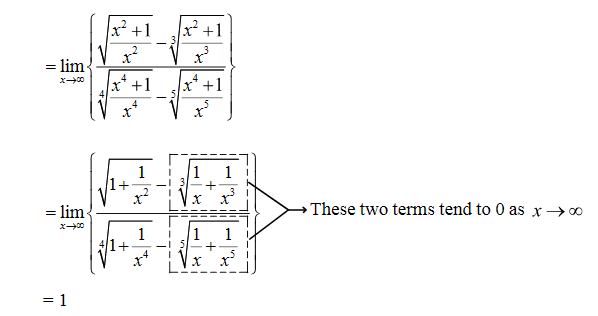(d) since the denominator is x–1, we can get a hint that the numerator

$(x{\rm{ \;}} + {\rm{\; }}{x^2} + {\rm{\; }}... + {x^n}){\rm{ \;}}-{\rm{\; }}n$

can be written as

$\left( {x{\rm{\;}}-{\rm{\;}}1} \right){\rm{\;}} + {\rm{ \;}}\left( {{x^2}-{\rm{\; }}1} \right){\rm{\;}} + {\rm{\; }}...{\rm{\;}} + {\rm{\;}}\left( {{x^n}-{\rm{ }}1} \right)$

so that

\begin{align}\mathop {\lim }\limits_{x \to 1} \frac{{\left( {x + {x^2} + ... + {x^n}} \right) - n}}{{x - 1}} &= \mathop {\lim }\limits_{x \to 1} \left\{ {\frac{{x - 1}}{{x - 1}} + \frac{{{x^2} - 1}}{{x - 1}} + ... + \frac{{{x^n} - 1}}{{x - 1}}} \right\}\\& = {\rm{\;}}1{\rm{\;}} + {\rm{\;}}2{\rm{\;}} + {\rm{\;}}3{\rm{\;}} + {\rm{\;}}... + {\rm{\;}}n\\ &= \frac{{n\left( {n + 1} \right)}}{2}\end{align}

Limits
grade 11 | Questions Set 1
Limits
Limits
grade 11 | Questions Set 2
Limits